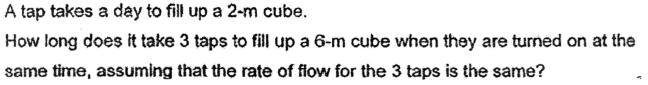# Questionpls help to solve this Q. thank you.

Method 1 :
2 x 2 x 2 = 8 (volume of 2-m cube)
6 x 6 x 6 = 216 (volume of 6-m cube)
216/8 = 27
27/3 = 9

Method 2 :
2 x 2 x 2 = 8 (volume of 2-m cube)
1 tap x 1 day ——- 8
3 taps x 1 day ——- 24
6 x 6 x 6 = 216 (volume of 6-m cube)
216/24 = 9

Ans : 9 days.